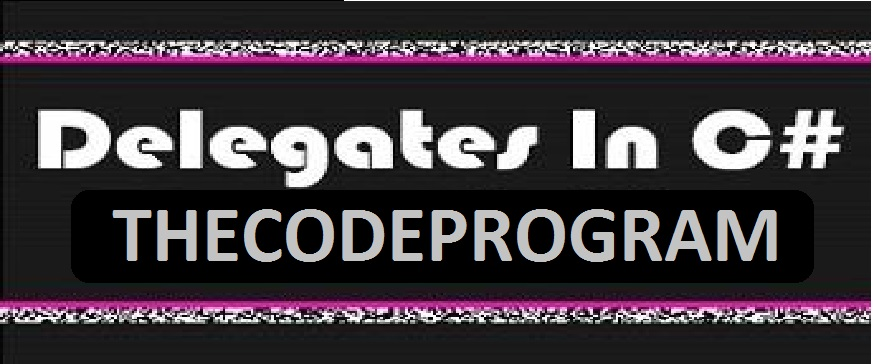09/07/2019 15

## Delegates in C#

We are gonna talk about delegates in C#. Our program will have much power and will be efficient with this objects.

Delegates in C# is similar to pointers in C and C++. A delegate is a reference type variable that holds the reference to a method. The reference can be changed at runtime.

We can use these classes to call external methods and so we can call back these external methods. We need to add the System. Delegate library to our project.

``````
using System.Delegate;
``````

## Declerating Delegate Objects

With delegate a method can be bounded to a referance. With doing this a delegate represent a method. So to call a method we use this delegate object. When we call this delegate, this method will be called back.

There is a delegate returns int and accepts string values declerated below

``````
delegate int delegate_object(string value);
``````

Here is the template of delegating:

``````
delegate variable_type delegate_name (function_parameters)
``````

## How to start a delegate ?

Before delegate started, it must be declerated with new keyword and must have relation with function. After declerating this delegate variable, calling this variables will go to this main function. Below code block have example about declerating delegate object to function.

``````
public delegate void print_function(string value);
yazdir func1 = new print_function("increase");
yazdir func2 = new print_function("decrease");
``````

Below code block have examples about declerating and calling delegate object. We can send parameter and it returns some value.

``````
using System;

delegate int my_delegate(int deger);
namespace delegate_example
{
class delegate_example
{
static int number = 33;
public static int increase_number(int val)
{
number += val;
return number;
}

public static int multiply(int val)
{
number *= val;
return number;
}
public static int get_number()
{
return number;
}

static void Main(string[] args)
{

my_delegate d1 =new my_delegate(increase_number);
my_delegate d2=new my_delegate(multiply);
//Pay Attention!!! We did not declare parameters here
//we will use these parameters with delegates below

d1(10); // we are gonna send parameters here
Console.WriteLine("Result :" + get_number());
d1(2);
Console.WriteLine("Result :" + get_number());
}
}
}

``````

Result of the code block above is :

``````
Result : 43
Result : 86
``````

## Multipling the delegate object

Delegate objects can combine with + sign. The delegate object that is concatenated calls the invoked functions concatenated. Only delegate objects of the same type can be joined. So int with int string with string bool vs. bool. delegate objects can be merged. The - sign is used to parse the component from the merged delegate object.

Sometimes multiplexing or decrementing operations can be used in the requesting processes within the software. This is termed multicasing. Let's make an example below.

``````
using System;

delegate int delegate_object(int n);
namespace delegate_application
{
class delegate
{
static int number =10;
public static int increase(int> val)
{
number += val;
return number;
}

public static int multply(int val)
{
number *= val;
return number;
}

public static int get_number()
{
return number;
}

static void Main(string[] args)
{
//declaring delegate objects
delegate_object d;
delegate_object d1 = new delegate_object(increase);
delegate_object d2 = new delegate_object(multply);

d = d1(5);
d += d2(5);

//making mathematical calculations above
// and writing result on the screen
Console.WriteLine("Result : " + get_number());

}
}
}
``````

First we equalized d1 to d and added 5 first result is 15. Then we multiplied this result with 5 with second delegate function and the result is the 75.

``````
Result : 75
``````

## Example of delegate usage

Now mates, Please lastly let me make a last example about delegates and finish the article.

``````
using System;
using System.IO;

namespace delegate_program
{
class delegate_app
{
static FileStream fs;
static StreamWriter sw;

// declare the delegate object
public delegate void print(string data);

//write on screen
public static void print_on_screen(string data)
{
Console.WriteLine("Result : " + data);
}

//write in a file
public static void print_in_file(string data)
{
fs = new FileStream ( "c:/file.txt" ,
FileMode.Append,FileAccess.Write);
sw = new StreamWriter(fs);
sw.WriteLine(data);
sw.Flush();
sw.Close();
fs.Close();
}

public static void send_text(print p)
{
p("TheCodeProgram");
}
static void Main(string[] args)
{
print y1 = new print(print_on_screen);
print y2 = new print(print_in_file);
send_text(y1);
send_text(y2);
}
}
}
``````

Result of the above code block is the below:

``````
Result : TheCodeProgram
``````

Now we know how to declare delegate objects and call it. We have made some examples about delegates.

We can use delegates to power up our program and make it more efficient.

Burak Hamdi TUFAN

Good works for everyone.